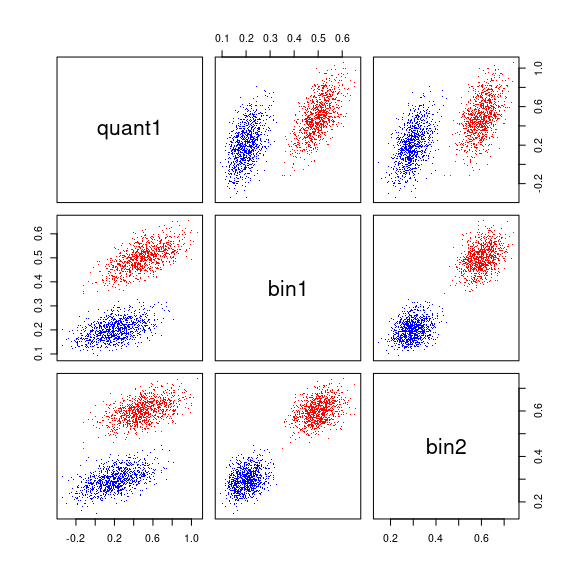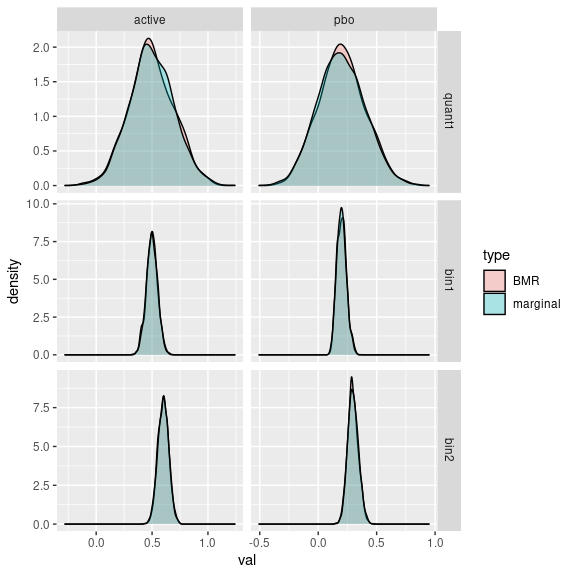# Bayesian Marginal Reconstruction

Suppose we are able to summarize the current state of scientific knowledge for the mean/proportion of each of several endpoints for a particular treatment using a distribution. The distribution for each endpoint may come from the elicitation of prior information from experts, some initial dataset or some combination of different sources of information. In many cases it will be difficult to arrive at a joint distribution. Only the marginal distribution of each endpoint will be calculated easily. This is particularly true when using externally published data where only marginal effects are known, and no patient level data is available. In general assuming independence for the distribution of the mean for each endpoint is not appropriate, as we would expect correlations in the distribution given that many endpoints are correlated. The method described here may be used to simulate from the approximate joint distribution given the marginal distribution and an individual level data set. The correlation structure within the individual level data is used to impute the joint distribution. The method also provides a way to simulate virtual trial data based on the marginal.

# A simulated example dataset

As an example, we simulate the following simple dataset with a continuous and two binary variables.

library(tboot)
set.seed(2020)
quant1  <- rnorm(200) + 1
bin1    <- ifelse( (.5*quant1 + .5*rnorm(200)) > .5, 1, 0)
bin2    <- ifelse( (.5*quant1 + .5*rnorm(200)) > .5, 1, 0)
simData <- data.frame(quant1, bin1, bin2)
head(simData)
##        quant1 bin1 bin2
## 1  1.37697212    0    0
## 2  1.30154837    1    1
## 3 -0.09802317    0    0
## 4 -0.13040590    0    0
## 5 -1.79653432    0    0
## 6  1.72057350    0    1

# Example

First, we create a list with simulations from the marginal distribution of each variable for two different treatments (active treatment and placebo).

marginal_active <-  list(quant1=rnorm(5000, mean=.5, sd=.2),
bin1=rbeta(5000, shape1 = 50,shape2=50),
bin2=rbeta(5000, shape1 = 60,shape2=40))
marginal_pbo <-  list(quant1=rnorm(5000, mean=.2, sd=.2),
bin1=rbeta(5000, shape1 = 20,shape2=80),
bin2=rbeta(5000, shape1 = 30,shape2=70))

We next need to use ‘tweights_bmr’ to calculate the correlation matrix from the data and get set for marginal reconstruction. The calculation uses a call to the ‘tweights’ function.

bmr_active <- tweights_bmr(dataset = simData, marginal = marginal_active)
## ----------------------------------------------------------------
## Optimization was successful. The weights have a sampling
## distribution with means close to the attempted target:
##                  quant1      bin1     bin2
## Achieved Mean 0.4968368 0.4992157 0.600457
## Target Mean   0.4968368 0.4992157 0.600457
## Maximum weight was:  0.04623098
## Data augmented with 1 sample(s) with independent variables.
## The final weight of the indpendent sample(s) was:  0.007398153
## ----------------------------------------------------------------
bmr_pbo <- tweights_bmr(dataset = simData, marginal = marginal_pbo)
## ----------------------------------------------------------------
## Optimization was successful. The weights have a sampling
## distribution with means close to the attempted target:
##                  quant1      bin1      bin2
## Achieved Mean 0.1969663 0.2003352 0.2997904
## Target Mean   0.1969663 0.2003352 0.2997904
## Maximum weight was:  0.02418729
## Data augmented with 1 sample(s) with independent variables.
## The final weight of the indpendent sample(s) was:  0.006562899
## ----------------------------------------------------------------

To simulate from the posterior we use ‘post_bmr’:

samples <- rbind(data.frame(trt="active", post_bmr(nsims=1e3, bmr_active)),
data.frame(trt="pbo", post_bmr(nsims=1e3, bmr_pbo)))
head(samples)
##      trt    quant1      bin1      bin2
## 1 active 0.4909751 0.4588719 0.5872279
## 2 active 0.1522832 0.3929919 0.4903635
## 3 active 0.3521943 0.4217331 0.5940661
## 4 active 0.1911470 0.4232380 0.5223984
## 5 active 0.4904320 0.5739046 0.5425915
## 6 active 0.6137868 0.5463136 0.6449981

The posterior samples show a correlations structure.

pairs(samples[,-1], col=ifelse(samples=="active","red","blue"), pch='.', cex=.5)Marginally the posterior samples are equivalent to the simulations used as input (i.e., in the ‘marginal’ parameter).

library(ggplot2)
pltdta=do.call(rbind, lapply(c("quant1","bin1", "bin2"),
function(nm) {
rbind(data.frame(type="BMR", var=nm, trt=samples$trt, val=samples[[nm]]), data.frame(type="marginal", var=nm, trt="active", val=marginal_active[[nm]]), data.frame(type="marginal", var=nm, trt="pbo", val=marginal_pbo[[nm]])) })) ggplot(pltdta, aes(fill=type, x=val)) + geom_density(alpha=.3) + facet_grid(var~trt, scales = "free")To simulate a random trial dataset using the parameters from a single draw of ‘post_bmr’ we use the tboot_bmr function. For example, to simulate 100 patients on active treatment: active_sample=tboot_bmr(nrow=100, weights_bmr=bmr_active) head(active_sample) ## quant1 bin1 bin2 ## 1 0.3944395 1 1 ## 2 -0.4321196 0 0 ## 3 -2.0566837 0 0 ## 4 -1.2648538 0 0 ## 5 -0.1767540 0 0 ## 6 -2.0566837 0 0 The underlying parameter mean for the simulation is an attribute: attr(active_sample, "post_bmr") ## quant1 bin1 bin2 ## 0.5927736 0.4617252 0.5994379 A more interesting example would be to simulate and analyze trial data. For example: #Manage any errors by assuming the pvalue failed to reach statistical #significance (i.e. pvalue is 1) but keep track of such errors. errorTrackGlobal=list() manageError=function(expr) { tryCatch(eval(quote(expr)), error=function(e){ errorTrackGlobal[[length(errorTrackGlobal)+1]] <<- e$message
return(1)
})
}

#create function to simulate and analyze one virtual trial
sim_and_analyze=function() {
active_sample=tboot_bmr(100, bmr_active)
pbo_sample=tboot_bmr(100, bmr_pbo)
data.frame(
p_quant1=manageError(t.test(active_sample$quant1,pbo_sample$quant1)$p.value), p_bin1=manageError(fisher.test(active_sample$bin1,pbo_sample$bin1)$p.value),
p_bin2=manageError(fisher.test(active_sample$bin2,pbo_sample$bin2)\$p.value)
)
}
#Simulate Pvalues
p_sim=do.call(rbind, replicate(100, sim_and_analyze(), simplify = FALSE))
head(errorTrackGlobal)
## list()
head(p_sim)
##       p_quant1     p_bin1     p_bin2
## 1 8.361678e-03 1.00000000 1.00000000
## 2 8.493083e-07 1.00000000 1.00000000
## 3 2.170874e-02 0.55474538 1.00000000
## 4 9.924969e-03 0.59836673 0.40612432
## 5 2.154656e-03 0.09505923 0.03519902
## 6 4.807536e-01 0.61658432 0.82775616

The pvalue matrix above may be analyzed, for example, using the gMCP package if multiple testing adjustments are needed.

# Methods

## The algorithm

To describe the algorithm, we use the following notation:

• $$k \in [1,2,...K]$$ is the endpoint index for $$K$$ endpoints.
• $$y_{k}$$ is a vector of length $$J_k$$ of simulations of the marginal distribution of the mean of $$k^{th}$$ endpoint.
• $$X$$ is a matrix of input data with columns for each endpoint. $$X_{.k}$$ is the vector of data for the $$k^{th}$$ endpoint.
• $$\hat{y}_k$$ is the mean of $$y_{k}$$.
• $$Q(y_{k}, p)$$ is the $$p^{th}$$ quantile of vector $$y_{k}$$.

The algorithm for ‘tweights_bmr()’ takes $$y_{k}$$ and $$X$$ as input and proceeds as follows:

1. Calculate $$\hat{y}_k$$.
2. Use tboot to calculate the weights ($$w$$) which would tilt $$X$$ such that the mean of $$w\cdot X_{.k} = \hat{y}_k$$ for all endpoints $$k$$.
3. Calculate the implied weighted correlation ($$\hat{C}$$) from using weights $$w$$ for $$X$$.

The algorithm for ‘post_bmr()’ takes the output from ‘tweights_bmr()’ and proceeds as follows:

1. Simulate $$Z \sim MultivariateNomal(mean=0, variance=\hat{C})$$
2. Simulate the posterior mean as $$y_k^* = Q(y_{k}, \Phi(Z_k))$$
3. Repeat steps 1 and 2 to generate more samples.

The algorithm for ‘tboot_bmr()’ takes the output from ‘tweights_bmr()’ and proceeds as follows:

1. Simulate the posterior mean $$\mu$$ as in the algorithm above.
2. Use tweights and tboot to simulate data with a mean of $$\mu$$. The option ‘Nindependent’ is always non-zero to help avoid errors. See the vignette on ‘tweights’ for more information on this option.

## Justifying the algorithm

The algorithm described above may be justified in several ways. First, it is heuristically plausible. One would expect at first thought that when two variables are correlated, a drug which influences one of the variables will most likely influence the other. Second, in some specific cases, the algorithm may be justified via Bayesian Asymptotics using the ‘Berstein Von-Misus’ theorem. This document will not attempt to fully work out this more theoretical approach.

## Considering the limits of ‘tboot_bmr’

The following considerations should be relevent when considering the use of ‘tboot_bmr:’

1. Is the relationship between variables found in the available individual level data generalizable to the treatment of interest? That is, if the individual data is tilted to reflect the expected mean of the treatment of interest, will the correlation be realistically similar to the correlation of variables in the treatment of interest. In general, it is expected that the assumptions of ‘tboot’ will be more believable than the assumption of independence.
2. Is the individual level data sample size large enough to make inference about correlation?
3. Did the information about each variable come from different trials? In such cases it may be argued that for large samples sizes the distribution should be independent.